# Methods for Fit Model and Discover Key Predictors with TreeNet® Classification

###### Note

This command is available with the Predictive Analytics Module. Click here for more information about how to activate the module.

TreeNet® models are an approach to solving classification and regression problems that are both more accurate and resistant to overfitting than a single classification or regression tree. A broad, general description of the process is that we begin with a small regression tree as an initial model. From that tree come residuals for every row in the data which become the response variable for the next regression tree. We build another small regression tree to predict the residuals from the first tree and compute the resulting residuals again. We repeat this sequence until an optimal number of trees with minimum prediction error is identified using a validation method. The resulting sequence of trees makes the TreeNet® Classification Model.

For the classification case, we can add some more mathematical detail for an analysis with a binary response and for an analysis with a multinomial response.

## Binary response

The creation of the model uses the following information:
• The response variable,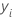, takes the following values: {-1, 1}.
• The initial fitted values for the calculation of the generalized residuals have the following form:

Where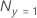is the number of events and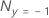is the number of nonevents.

The model creation also uses the following inputs from the analyst:
Input Symbol
learn rate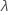sampling ratemaximum number of terminal nodes per tree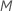number of treesThe process has the following general steps for growing the jth tree, j=1,...,J:
1. Draw a random sample of size s * N from the training data, where N is the number of rows in the training data.
2. Calculate the generalized residuals, gi, j, for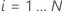: where

and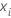is a vector that represents the ith row of the predictor values in the training data.

3. Fit a regression tree with at most M terminal nodes to the generalized residuals. The tree partitions the observations into at most M mutually exclusive groups.
4. For the mth terminal node in the regression tree, calculate the within-node updates for fitted values from the previous tree as follows:
where
TermDescription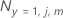number of events in terminal node m at tree j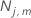number of cases in terminal node m at tree j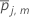arithmetic mean of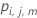for all cases in terminal node m at tree j
5. Shrink the within-node updates by the learning rate and apply the values to get the updated fitted values, fj(xi):
6. Repeat steps 1-5 for each of the J trees in the analysis.

## Multinomial response

For a multinomial response with K levels, the analysis fits a tree to each level of the response variable at each iteration. The initial fitted values for the calculation of the generalized residuals for one of the trees has the following form:

where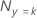is the number of cases where the response value is k and N is the number of rows in the training data.

The model creation also uses the following inputs from the analyst:
Input Symbol
learn ratesampling ratemaximum number of terminal nodes per treenumber of treesThe calculation of the probabilities from the fits accounts for the dependent nature of these trees. Otherwise, the process is substantially the same as for the binary case.

1. Draw a random sample of size s * N from the training data, where N is the number of rows in the training data set.
2. Calculate the generalized residuals, gi, j, k for,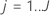, the number of trees in the analysis, and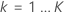, the number of levels of the response variable:

where

andis a vector that represents the ith row of the predictor values in the training data set.

For example, the probability for an outcome coded as 1 from a multinomial response with 3 levels has the following form:
where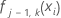is the fit for the ith row at the j–1 tree for the kth level of the response variable.
3. Fit a regression tree with at most M terminal nodes to the generalized residuals. The tree partitions the observations into at most M mutually exclusive groups.
4. For the mth terminal node in the jth regression tree, calculate the within-node updates for fitted values from the previous tree as follows:

where

TermDescription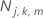number of cases for outcome k in terminal node m at tree jnumber of cases in terminal node m at tree j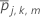arithmetic mean of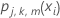for all cases in terminal node m at tree j.
5. Shrink the within-node updates by the learning rate and apply the values to get the updated fitted values, fj, k, m(xi):
6. Repeat steps 1-5 for each of the J trees in the analysis and for each of the K levels of the response variable.
By using this site you agree to the use of cookies for analytics and personalized content.  Read our policy